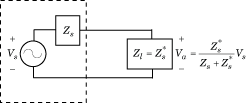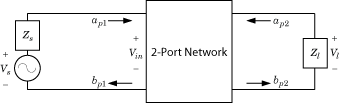# s2tf

Convert S-parameters of 2-port network to voltage or power-wave transfer function

## Syntax

``tf = s2tf(s_params)``
``tf = s2tf(s_params,z0,zs,zl)``
``tf = s2tf(s_params,z0,zs,zl,option)``
``tf = s2tf(hs)``
``tf = s2tf(hs,zs,zl)``
``tf = s2tf(hs,zs,zl,option)``

## Description

example

````tf = s2tf(s_params)` converts the scattering parameters of a 2-port network to the voltage transfer function of the network. ```
````tf = s2tf(s_params,z0,zs,zl)` calculates the voltage transfer function using the reference impedance `z0`, source impedance `zs`, and load impedance `zl`.`tf = s2tf(s_params,z0,zs,zl,option)` calculates the voltage or power-wave transfer function using the method specified by `option`.```
````tf = s2tf(hs)` converts the 2-port S-parameter object, `hs`, into the voltage transfer function of the network. ```
````tf = s2tf(hs,zs,zl)` calculates the voltage transfer function using the source impedance `zs`, and load impedance `zl`.```
````tf = s2tf(hs,zs,zl,option)` calculates the voltage or power-wave transfer function using the method specified by `option`.```

## Examples

collapse all

Calculate the voltage transfer function of an S-parameter array.

```ckt = read(rfckt.passive,'passive.s2p'); sparams = ckt.NetworkData.Data; tf = s2tf(sparams)```
```tf = 202×1 complex 0.9964 - 0.0254i 0.9960 - 0.0266i 0.9956 - 0.0284i 0.9961 - 0.0290i 0.9960 - 0.0301i 0.9953 - 0.0317i 0.9953 - 0.0334i 0.9952 - 0.0349i 0.9949 - 0.0367i 0.9946 - 0.0380i ⋮ ```

Calculate the voltage transfer function of a S-parameters object using `s2tf` function.

```sparams = sparameters('passive.s2p'); tf = s2tf(sparams)```
```tf = 202×1 complex 0.9964 - 0.0254i 0.9960 - 0.0266i 0.9956 - 0.0284i 0.9961 - 0.0290i 0.9960 - 0.0301i 0.9953 - 0.0317i 0.9953 - 0.0334i 0.9952 - 0.0349i 0.9949 - 0.0367i 0.9946 - 0.0380i ⋮ ```

## Input Arguments

collapse all

2-port S-parameters, specified as an RF Toolbox™ S-parameter object.

Scattering parameters, specified as a complex 2-by-2-by-M array where M represents the number of frequency points of the S-parameters.

Reference impedance of S-parameters, specified in ohms.

Source impedance of S-parameters, specified in ohms.

Load impedance of S-parameters, specified in ohms.

Transfer function type, specified as an integer equal to `1`, `2`, or `3`.

• `1` — The transfer function is the gain from the incident voltage, Va, to the output voltage for arbitrary source and load impedances:

`$tf=\frac{{V}_{l}}{{V}_{a}}$`

The following figure shows how to compute Va from the source voltage Vs:For the S-parameters and impedance values, the transfer function is:

`$tf=\frac{\left({Z}_{s}+{Z}_{s}^{*}\right)}{{Z}_{s}^{*}}\frac{{S}_{21}\left(1+{\Gamma }_{l}\right)\left(1-{\Gamma }_{s}\right)}{2\left(1-{S}_{22}{\Gamma }_{l}\right)\left(1-{\Gamma }_{in}{\Gamma }_{s}\right)}$`

where:

`$\begin{array}{c}{\Gamma }_{l}=\frac{{Z}_{l}-{Z}_{o}}{{Z}_{l}+{Z}_{o}}\\ {\Gamma }_{s}=\frac{{Z}_{s}-{Z}_{o}}{{Z}_{s}+{Z}_{o}}\\ {\Gamma }_{in}={S}_{11}+\left({S}_{12}{S}_{21}\frac{{\Gamma }_{l}}{\left(1-{S}_{22}{\Gamma }_{l}\right)}\right)\end{array}$`

The following equation shows how the preceding transfer function is related to the transducer gain computed by the `powergain` function:

`${G}_{T}={|tf|}^{2}\frac{\mathrm{Re}\left({Z}_{l}\right)}{{|{Z}_{l}|}^{2}}\frac{{|{Z}_{s}|}^{2}}{\mathrm{Re}\left({Z}_{s}\right)}$`

Notice that if Zl and ZS are real, ${G}_{T}={|tf|}^{2}\frac{{Z}_{s}}{{Z}_{l}}$.

• `2` — The transfer function is the gain from the source voltage to the output voltage for arbitrary source and load impedances:

`$tf=\frac{{V}_{l}}{{V}_{s}}=\frac{{S}_{21}\left(1+{\Gamma }_{l}\right)\left(1-{\Gamma }_{s}\right)}{2\left(1-{S}_{22}{\Gamma }_{l}\right)\left(1-{\Gamma }_{in}{\Gamma }_{s}\right)}$`

You can use this option to compute the transfer function $\frac{{V}_{{}_{L}}}{{V}_{in}}$ by setting `zs` to `0`. This setting means that Γs = –1 and Vin = Vs.

• `3` — The transfer function is the power-wave gain from the incident power wave at the first port to the transmitted power wave at the second port:

`$tf=\frac{{b}_{p2}}{{a}_{p1}}=\frac{\sqrt{\mathrm{Re}\left({Z}_{l}\right)\mathrm{Re}\left({Z}_{s}\right)}}{{Z}_{l}}\frac{{S}_{21}\left(1+{\Gamma }_{l}\right)\left(1-{\Gamma }_{s}\right)}{\left(1-{S}_{22}{\Gamma }_{l}\right)\left(1-{\Gamma }_{in}{\Gamma }_{s}\right)}$`

## Output Arguments

collapse all

Voltage transfer function, returned as a vector of doubles.

Complex Number Support: Yes

## Algorithms

The following figure shows the setup for computing the transfer function, along with the impedances, voltages, and the power waves used to determine the gain.The function uses the following voltages and power waves for calculations:

• Vl is the output voltage across the load impedance.

• Vs is the source voltage.

• Vin is the input voltage of the 2-port network.

• ${a}_{p1}$ is the incident power wave, equal to $\frac{{V}_{s}}{2\sqrt{\mathrm{Re}\left({Z}_{s}\right)}}$.

• ${b}_{p2}$ is the transmitted power wave, equal to $\frac{\sqrt{\mathrm{Re}\left({Z}_{l}\right)}}{{Z}_{l}}{V}_{l}$.

 Gonzalez, Guillermo. Microwave Transistor Amplifiers: Analysis and Design. 2nd ed, Prentice Hall, 1997.